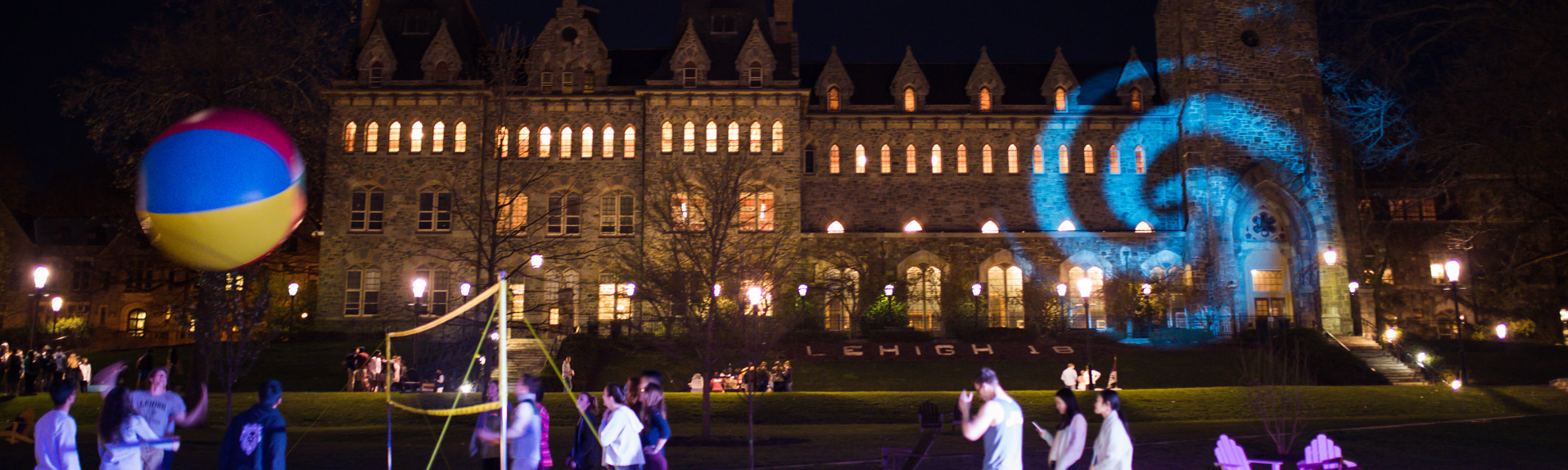# Dr. Xianyi Zeng, Mechanical Engineering & Math Seminar | Mechanical Engineering & Mechanics

Friday, November 16, 2018 at 4:10pm

Packard Laboratory, 466
19 Memorial Dr W, Bethlehem, PA 18015

Coupling Embedded-Boundary and ALE Methods for Multi-Material Flow Computations

ABSTRACT

We present a computational framework that combines the embedded boundary method and the arbitrary Lagrangian-Eulerian (ALE) method for multi-material flow computations of compressible fluids. This methodology is capable of handling geometrically complex material interfaces on moving grids and is suitable for multi-material flow computations in the context of ALE shock hydrodynamics. Specifically, we use a variational multiscale stabilized (VMS) finite element method to update the fluid state of each material, and define ghost values to enforce the transmission condition at the embedded material interface, which is captured using a level-set approach. Two different strategies to populate ghost values are considered and compared, namely simple constant extrapolation and a multi-material Riemann solver. The accuracy and conservation properties of both ghost value population strategies are evaluated using benchmark shock tube problems and blast/bubble interaction problems. The method is then extended to solve multi-fluid problems with more than two fluids such as the triple-point problem, where multiple material interfaces are captured by the projected level set method; and it is also extended for numerical simulation of a liquid-gas-solid interaction problem, where body-fitted grids are used to track the multi-fluid/solid interface and an embedded boundary separates the liquid from the gas.

BIOSKETCH

Dr. Xianyi Zeng received his B.S. in mathematics from Peking University, China, and then the Ph.D. in computational mathematics from Stanford University in 2012. After working on computational mechanics as a Postdoc at Duke University, he joined the Department of Mathematical Sciences and Computational Science Program of The University of Texas at El Paso in 2016. Dr. Zeng has broad research interests in numerical partial differential equations and their applications in physics, mechanical engineering, and biological sciences, as well as their interfacing with data and statistical sciences, such as curve/surface fitting, stochastic processes, and uncertainty quantification. His current research focuses are multi-material flow computations and multi-physics problems, numerical modeling of tumor growth, and novel discretization methods for hyperbolic conservation laws.

Topic
Target Audience
Department
Mathematics Department, Mechanical Engineering and Mechanics Department
Contact Information

Barbara McGuire, bcm208@lehigh.edu